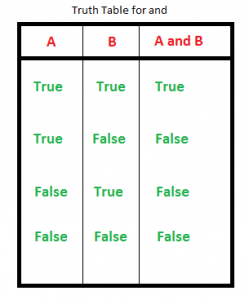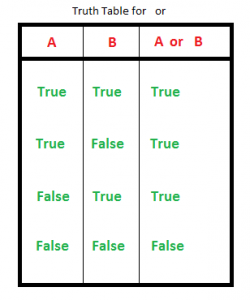# Python Keywords

• Difficulty Level : Easy
• Last Updated : 12 Oct, 2021

Python Keywords: Introduction

Keywords in Python are reserved words that can not be used as a variable name, function name, or any other identifier.

Attention geek! Strengthen your foundations with the Python Programming Foundation Course and learn the basics.

To begin with, your interview preparations Enhance your Data Structures concepts with the Python DS Course. And to begin with your Machine Learning Journey, join the Machine Learning - Basic Level Course

## List of all keywords in Python

We can also get all the keyword names using the below code.

## Python3

 `# Python code to demonstrate working of iskeyword()`` ` `# importing "keyword" for keyword operations``import` `keyword`` ` `# printing all keywords at once using "kwlist()"``print``(``"The list of keywords is : "``)``print``(keyword.kwlist)`

Output:

The list of keywords is :

[‘False’, ‘None’, ‘True’, ‘and’, ‘as’, ‘assert’, ‘async’, ‘await’, ‘break’, ‘class’, ‘continue’, ‘def’, ‘del’, ‘elif’, ‘else’, ‘except’, ‘finally’, ‘for’, ‘from’, ‘global’, ‘if’, ‘import’, ‘in’, ‘is’, ‘lambda’, ‘nonlocal’, ‘not’, ‘or’, ‘pass’, ‘raise’, ‘return’, ‘try’, ‘while’, ‘with’, ‘yield’]

Let’s discuss each keyword in detail with the help of good examples.

## True, False, None

• True: This keyword is used to represent a boolean true. If a statement is true, “True” is printed.
• False: This keyword is used to represent a boolean false. If a statement is false, “False” is printed.
• None: This is a special constant used to denote a null value or a void. It’s important to remember, 0, any empty container(e.g empty list) does not compute to None.
It is an object of its datatype – NoneType. It is not possible to create multiple None objects and can assign them to variables.

## Python3

 `print``(``False` `=``=` `0``)``print``(``True` `=``=` `1``)`` ` `print``(``True` `+` `True` `+` `True``)``print``(``True` `+` `False` `+` `False``)`` ` `print``(``None` `=``=` `0``)``print``(``None` `=``=` `[])`
Output
```True
True
3
1
False
False
```

## and, or, not, in, is

• and: This a logical operator in python. “and” Return the first false value. If not found return last. The truth table for “and” is depicted below.3 and 0 returns 0

3 and 10 returns 10

10 or 20 or 30 or 10 or 70 returns 10

The above statements might be a bit confusing to a programmer coming from a language like C where the logical operators always return boolean values(0 or 1). Following lines are straight from the python docs explaining this:

The expression x and y first evaluates x; if x is false, its value is returned; otherwise, y is evaluated and the resulting value is returned.

The expression x or y first evaluates x; if x is true, its value is returned; otherwise, y is evaluated and the resulting value is returned.

Note that neither and nor or restrict the value and type they return to False and True, but rather return the last evaluated argument. This is sometimes useful, e.g., if s is a string that should be replaced by a default value if it is empty, the expression s or ‘foo’ yields the desired value. Because not has to create a new value, it returns a boolean value regardless of the type of its argument (for example, not ‘foo’ produces False rather than ”.)

• or: This a logical operator in python. “or” Return the first True value.if not found return last. The truth table for “or” is depicted below.3 or 0 returns 3

3 or 10 returns 3

0 or 0 or 3 or 10 or 0 returns

• not: This logical operator inverts the truth value. The truth table for “not” is depicted below.
• in: This keyword is used to check if a container contains a value. This keyword is also used to loop through the container.
• is: This keyword is used to test object identity, i.e to check if both the objects take the same memory location or not.

## Python

 `# showing logical operation``# or (returns True)``print``(``True` `or` `False``)`` ` `# showing logical operation``# and (returns False)``print``(``False` `and` `True``)`` ` `# showing logical operation``# not (returns False)``print``(``not` `True``)`` ` `# using "in" to check``if` `'s'` `in` `'geeksforgeeks'``:``    ``print``(``"s is part of geeksforgeeks"``)``else``:``    ``print``(``"s is not part of geeksforgeeks"``)`` ` `# using "in" to loop through``for` `i ``in` `'geeksforgeeks'``:``    ``print``(i, end``=``" "``)`` ` `print``(``"\r"``)`` ` `# using is to check object identity``# string is immutable( cannot be changed once allocated)``# hence occupy same memory location``print``(``' '` `is` `' '``)`` ` `# using is to check object identity``# dictionary is mutable( can be changed once allocated)``# hence occupy different memory location``print``({} ``is` `{})`

Output:

```True
False
False
s is part of geeksforgeeks
g e e k s f o r g e e k s
True
False```

## Iteration Keywords – for, while, break, continue

• for: This keyword is used to control flow and for looping.
• while: Has a similar working like “for”, used to control flow and for looping.
• break: “break” is used to control the flow of the loop. The statement is used to break out of the loop and passes the control to the statement following immediately after loop.
• continue: “continue” is also used to control the flow of code. The keyword skips the current iteration of the loop but does not end the loop.

## Python3

 `# Using for loop``for` `i ``in` `range``(``10``):`` ` `    ``print``(i, end ``=` `" "``)``     ` `    ``# break the loop as soon it sees 6``    ``if` `i ``=``=` `6``:``        ``break``     ` `print``()``     ` `# loop from 1 to 10``i ``=` `0``while` `i <``10``:``     ` `    ``# If i is equals to 6,``    ``# continue to next iteration``    ``# without printing``    ``if` `i ``=``=` `6``:``        ``i``+``=` `1``        ``continue``    ``else``:``        ``# otherwise print the value``        ``# of i``        ``print``(i, end ``=` `" "``)``         ` `    ``i ``+``=` `1`
Output
```0 1 2 3 4 5 6
0 1 2 3 4 5 7 8 9 ```

## Conditional keywords – if, else, elif

• if : It is a control statement for decision making. Truth expression forces control to go in “if” statement block.
• else : It is a control statement for decision making. False expression forces control to go in “else” statement block.
• elif : It is a control statement for decision making. It is short for “else if

## Python3

 `# Python program to illustrate if-elif-else ladder``#!/usr/bin/python`` ` `i ``=` `20``if` `(i ``=``=` `10``):``    ``print` `(``"i is 10"``)``elif` `(i ``=``=` `20``):``    ``print` `(``"i is 20"``)``else``:``    ``print` `(``"i is not present"``)`
Output
```i is 20
```

## def

def keyword is used to declare user defined functions.

## Python3

 `# def keyword``def` `fun():``    ``print``(``"Inside Function"``)``     ` `fun()`
Output
```Inside Function
```

## Return Keywords – Return, Yield

• return : This keyword is used to return from the function.
• yield : This keyword is used like return statement but is used to return a generator.

## Python3

 `# Return keyword``def` `fun():``    ``S ``=` `0``     ` `    ``for` `i ``in` `range``(``10``):``        ``S ``+``=` `i``    ``return` `S`` ` `print``(fun())`` ` `# Yield Keyword``def` `fun():``    ``S ``=` `0``     ` `    ``for` `i ``in` `range``(``10``):``        ``S ``+``=` `i``        ``yield` `S`` ` `for` `i ``in` `fun():``    ``print``(i)`
Output
```45
0
1
3
6
10
15
21
28
36
45
```

## class

class keyword is used to declare user defined classes.

## Python3

 `# Python3 program to``# demonstrate instantiating``# a class`` ` ` ` `class` `Dog:``     ` `    ``# A simple class``    ``# attribute``    ``attr1 ``=` `"mammal"``    ``attr2 ``=` `"dog"`` ` `    ``# A sample method``    ``def` `fun(``self``):``        ``print``(``"I'm a"``, ``self``.attr1)``        ``print``(``"I'm a"``, ``self``.attr2)`` ` `# Driver code``# Object instantiation``Rodger ``=` `Dog()`` ` `# Accessing class attributes``# and method through objects``print``(Rodger.attr1)``Rodger.fun()`
Output
```mammal
I'm a mammal
I'm a dog
```

Note: For more information, refer to our Python Classes and Objects Tutorial .

## With

with keyword is used to wrap the execution of block of code within methods defined by context manager. This keyword is not used much in day to day programming.

## Python3

 `# using with statement``with ``open``(``'file_path'``, ``'w'``) as ``file``:``    ``file``.write(``'hello world !'``)`

## as

as keyword is used to create the alias for the module imported. i.e giving a new name to the imported module. E.g import math as mymath.

## Python3

 `import` `math as gfg`` ` `print``(gfg.factorial(``5``))`
Output
```120
```

## pass

pass is the null statement in python. Nothing happens when this is encountered. This is used to prevent indentation errors and used as a placeholder.

## Python3

 `n ``=` `10``for` `i ``in` `range``(n):``     ` `# pass can be used as placeholder``# when code is to added later``pass`

## Lambda

Lambda keyword is used to make inline returning functions with no statements allowed internally.

## Python3

 `# Lambda keyword``g ``=` `lambda` `x: x``*``x``*``x`` ` `print``(g(``7``))`
Output
```343
```

## Import, From

• import : This statement is used to include a particular module into current program.
• from : Generally used with import, from is used to import particular functionality from the module imported.

## Python3

 `# import keyword``import` `math``print``(math.factorial(``10``))`` ` `# from keyword``from` `math ``import` `factorial``print``(factorial(``10``))`
Output
```3628800
3628800
```

## Exception Handling Keywords – try, except, raise, finally, and assert

• try : This keyword is used for exception handling, used to catch the errors in the code using the keyword except. Code in “try” block is checked, if there is any type of error, except block is executed.
• except : As explained above, this works together with “try” to catch exceptions.
• finally : No matter what is result of the “try” block, block termed “finally” is always executed.
• raise: We can raise an exception explicitly with the raise keyword
• assert: This function is used for debugging purposes. Usually used to check the correctness of code. If a statement is evaluated to be true, nothing happens, but when it is false, “AssertionError” is raised. One can also print a message with the error, separated by a comma.

## Python3

 `# initializing number``a ``=` `4``b ``=` `0`` ` `# No exception Exception raised in try block``try``:``    ``k ``=` `a``/``/``b ``# raises divide by zero exception.``    ``print``(k)`` ` `# handles zerodivision exception``except` `ZeroDivisionError:``    ``print``(``"Can't divide by zero"``)`` ` `finally``:``    ``# this block is always executed``    ``# regardless of exception generation.``    ``print``(``'This is always executed'``)`` ` `# assert Keyword  ``# using assert to check for 0``print` `(``"The value of a / b is : "``)``assert` `b !``=` `0``, ``"Divide by 0 error"``print` `(a ``/` `b)`

Output

```Can't divide by zero
This is always executed
The value of a / b is :
AssertionError: Divide by 0 error```

Note: For more information refer to our tutorial Exception Handling Tutorial in Python.

## del

del is used to delete a reference to an object. Any variable or list value can be deleted using del.

## Python3

 `my_variable1 ``=` `20``my_variable2 ``=` `"GeeksForGeeks"`` ` `# check if my_variable1 and my_variable2 exists``print``(my_variable1)``print``(my_variable2)`` ` `# delete both the variables``del` `my_variable1``del` `my_variable2`` ` `# check if my_variable1 and my_variable2 exists``print``(my_variable1)``print``(my_variable2)`

Output

```20
GeeksForGeeks
NameError: name 'my_variable1' is not defined```

## Global, Nonlocal

• global: This keyword is used to define a variable inside the function to be of a global scope.
• non-local : This keyword works similar to the global, but rather than global, this keyword declares a variable to point to variable of outside enclosing function, in case of nested functions.

## Python3

 `# global variable``a ``=` `15``b ``=` `10`` ` `# function to perform addition``def` `add():``    ``c ``=` `a ``+` `b``    ``print``(c)`` ` `# calling a function``add()`` ` `# nonlocal keyword``def` `fun():``    ``var1 ``=` `10`` ` `    ``def` `gun():``        ``# tell python explicitly that it``        ``# has to access var1 initialized``        ``# in fun on line 2``        ``# using the keyword nonlocal``        ``nonlocal var1``         ` `        ``var1 ``=` `var1 ``+` `10``        ``print``(var1)`` ` `    ``gun()``fun()`
Output
```25
20
```

Note: For more information, refer to our Global and local variables tutorial in Python.

This article is contributed by Manjeet Singh(S. Nandini). If you like GeeksforGeeks and would like to contribute, you can also write an article using write.geeksforgeeks.org or mail your article to review-team@geeksforgeeks.org. See your article appearing on the GeeksforGeeks main page and help other Geeks.